# Can we really measure the velocity of light?

Definition of velocity

Is there any easier concept to learn in Physics than velocity? Take a body going from A to B, measure the distance between these two points and divide it by the time taken to complete the route; this is what is meant by velocity of that body. Quite simple. But, often, important issues lie concealed in the apparent simplicity of many physical definitions; velocity is one of them. To measure it, two clocks positioned at two points separated in space, say clock A and clock B, are needed. If any meaningful velocity measurement is to be done at all, these two clocks must be synchronized. Consider what would happen if this was not true. For instance, if clock B lagged 10 seconds behind clock A, and the body took exactly 10 seconds to go from A to B, we would end up with an unfeasible, infinite velocity! Once the need for synchronization has been established, it makes sense to ask how it can be carried out. Two possible ways come to mind. In the first, clock B is taken at position A and synchronized with clock A; then it is transported back to position B. After this operation the two clocks should be synchronized. But, are they, really? From one of the most successful theories of the 20th centuries, Special Relativity, we learn that any traveling clock slows down, compared to a stationary one. When clock B is transported from A to B it will, unavoidably, decrease its pace; once B is reached its ticking will equal the ticking of clock A, but the two clocks will have lost their synchronization. The second synchronization procedure uses light signals. When a time tA=0 is read at clock A, a signal having constant velocity c is sent towards B. Later the signal reaches B, after an amount of time Δt /c, (dAB is the distance between A and B), and clock B sets its time to tB= dAB /c. At this point the clocks are synchronized. The problem with this second synchronization procedure is that one cannot be sure about the signal velocity, c, because two synchronized clocks are required to measure velocity, but it is the velocity itself which is used to synchronize clocks! An unavoidable logical circularity appears to plague the second synchronization procedure.

It is, thus, clear that the concept of velocity is strongly interlaced with synchronization of distant clocks. This might come as a surprise to many Physics students, who have considered velocity one of the well-established ideas of Mechanics. As a matter of fact, philosophers rather than physicists have started all major objections to the definition of velocity. Jules Henri Poincaré (1854-1912), better known for its outstanding mathematical contributions to Physics and Engineering, has been the first to seriously criticize time and clocks synchronization.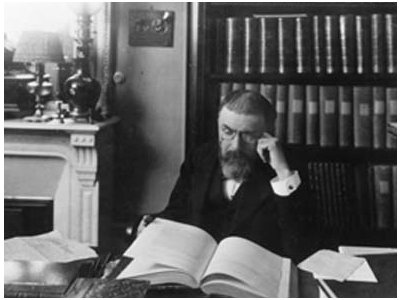Figure 1: The French scientist and mathematician Jules Henri Poincaré (1854-1912), has been the first to seriously criticize time and clocks synchronization.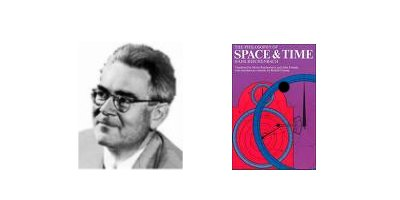Figure 2: Hans Reichenbach (1891-1953) and his influential book The Philosophy of Space and Time.

Another prominent character joining the debate was Hans Reichenbach (1891-1953). In 1928 he wrote an influential book titled The Philosophy of Space and Time, in which the fundamental notions of space, geometry, time and synchronization were discussed at length. Among them we find a thorough discussion on the velocity of light and the synchronization of distant clocks.

Clocks synchronization, one-way and two-way velocity of light

The conceptual difficulty hidden in the synchronization of distant clocks was quite clear to the creator of Special Relativity himself, Albert Einstein. In his celebrated article of 1905, On the Electrodynamics of Moving Bodies, the famous scientist wrote:

“If at the point A of space there is a clock, an observer at A can determine the time values of events in the immediate proximity of A by finding the positions of the hands which are simultaneous with these events. If there is at the point B of space another clock in all respects resembling the one at A, it is possible for an observer at B to determine the time values of events in the immediate neighborhood of B. But it is not possible without further assumption to compare, in respect of time, an event at A with an event at B. We have so far defined only an ``A time'' and a ``B time.'' We have not defined a common ``time'' for A and B, for the latter cannot be defined at all unless we establish by definition that the ``time'' required by light to travel from A to B equals the ``time'' it requires to travel from B to A.”

Einstein’s synchronization is essentially the synchronization through light signals described before. The time read at clock B is never actually read, it is only assigned conventionally. As previously seen, there is a logical impossibility to establish the velocity taken by the signal to travel from A to B. Einstein, therefore, assumes that light travels from A to B with the same speed used to travel from B to A. Speeds being the same, duration times will, accordingly, be the same. What emerges from Einstein’s discussion is a precise statement about the one-way velocity of light, i.e. the velocity measured in going from A to B or from B to A, using two distant clocks. This has to be weighted against the two-way velocity of light, which only requires one clock. To measure this second type of velocity one sends a light signal towards clock B, where it is instantly reflected towards clock A. The two-way velocity is simply obtained dividing twice the distance dAB by the total time, as measured by clock A. This two-way velocity is, thus, an average speed, although it has experimentally been proven that it maintains the same value, irrespective of its angular direction. It is normally indicated as c. In Special Relativity it is the one-way velocity of light to be considered a constant quantity. This is, in fact, one of Einstein postulates. In other words the one-way velocity of light, together with clock synchronization, is not found experimentally, but it is arbitrarily chosen, it is postulated. Reichenbach has criticized Einstein’s synchronization and has, conveniently, introduced a synchronization parameter, ε, to describe the arbitrary choice made in the theory (see Box 1). Choosing ε is equivalent to selecting a specific value for the one-way velocity of light. This parameter varies between (but not including) 0 and 1, with ε=0.5 corresponding to a one-way velocity equal to c. The extraordinary discovery found by a group of philosophers of science, later named the conventionalists, is that all experimental verifications of Special Relativity do not depend on ε. Any well-established and verified consequence of the theory, like time dilation, length contraction or, even, the celebrated E=mc2 formula, can be proved either by selecting ε=0.5, like done by Einstein, or by selecting any other value like, for instance, ε=0.2. Thus, the conventionalist thesis decisively asserts that an inescapable element of human choice is forever contained in the theory of Special Relativity. No matter how hard scientists have tried and will try to envisage experimental tests to rule out choices of ε different from 0.5, such experiments are always doomed to failure (Box 2). Physicists have never liked the thought that a quantity as important and universal as the velocity of light could depend on any sort of subjective human choice. Several of them have done their best to prove philosophers wrong but, alas, no decisive result has been produced yet.

Experimental measurements of the velocity of light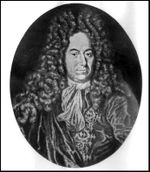Figure 3: The Danish astronomer Ole Rømer (1644-1710) is considered to be the first scientist to have successfully measured the velocity of light. His method considered anomalies in Jupiter's moons observed eclipses.

Ever since the finitude of light speed was first questioned and tested by the Danish astronomer Ole Rømer (1644-1710) in 1676, a multitude of methods to measure it more and more accurately have been suggested and carried out. There is simply no time to review all these methods in this article. It is, rather, more instructive to consider a couple of experimental measurements important for historical reasons and because, for a long time, they have given the impression that the one-way velocity of light is a perfectly measurable physical quantity. We are referring here to Rømer’s and Bradley’s measurements.

Let us describe Rømer’s measurement, first (see Figure 4).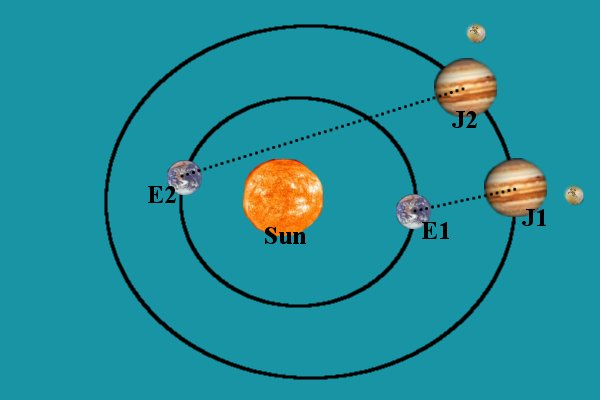Figure 4: Rømer's method of measuring the velocity of light. Jupiter's speed around the Sun is much slower than the Earth's speed. Thus, Jupiter's positions, J1 and J2, are close compared to the Earth's positions, E1 and E2, during half a revolution of the Earth. Light coming from Io will, therefore, take a longer time to traverse distance J2-E2, than distance J1-E1. The observed differences in Io's eclipses times can be explained using the additional time required by light to travel across a longer distance.

It was known for a long time that eclipses by Jupiter satellites appeared to occur at irregular times throughout the year. In particular the time interval between two consecutive occultations of Io, one of its biggest and most luminous moons, appeared to be stretching when the Earth moved from its closest to its farthest position with respect to Jupiter. Rømer correctly interpreted this anomaly by assuming that light had a finite speed. The observed differences were ultimately caused by the additional time light takes to traverse the Earth’s orbit. Although the value found in 1676 was not very accurate, mainly because it was difficult to estimate with precision the Earth’s orbit, later measurements have confirmed for c a value very close to the one currently adopted by the world scientific community. The velocity found with the method just described seems to be a real one-way velocity. Only one clock is needed to measure it, thus eliminating the synchronization problem, because consecutive measurements of Io’s eclipses are made using the same observatory on Earth. But this single clock is, in fact, traveling from one point to the next on the Earth’s orbit. Therefore is a moving clock and, as such, is subject to the slowing down predicted by Special Relativity. It can be shown rigorously that the speed measured in this way is equivalent to a two-way velocity. For many decades Rømer’s method was thought to be an effective way of measuring the one-way velocity of light, but there is now consensus among the experts in the field that this really is simply not true.

Let us turn, next, to Bradley’s method.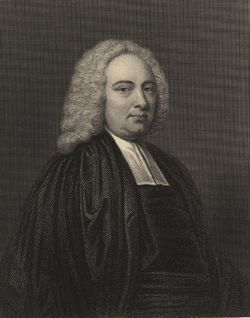Figure 5: James Bradley (1693-1762) was appointed Astronomer Royal in 1742. His major scientific contribution has been the discovery of light aberration.

James Bradley (1693–1762), English scientist appointed Astronomer Royal in 1742, discovered that he had to change the inclination of his telescope throughout the year, if he wanted to observe any distant star. This phenomenon can be observed using any optical telescope and it is known as star aberration. Bradley understood that aberration is connected to the velocity of light, and presented his detailed, correct explanation to the Royal Society in 1729. An effective way to learn and understand it goes through the comparison with a straw in the rain (see Figure 6).Figure 6: Light aberration can be explained through the comparison with raindrops falling through a straw. a) When the straw is still every raindrop entering its top will, eventually, come out of its bottom; b) if the straw moves with constant velocity to the left, every drop entering its top will hit the straw's wall, they will never emerge from the straw's bottom; c) raindrops can again come out of the straw if this is tilted of a certain angle.

In a straw kept still and vertical, all drops getting into its top will eventually come out of its bottom. If the straw is moving horizontally, though, a drop getting through its top can potentially hit its walls, thus never making it through its bottom. The horizontal speed, v, (and, obviously, the straw’s section) is key in determining whether the rain will traverse or be trapped in the straw. There is, though, a way to allow all drops to traverse the straw’s length, irrespective of its section or velocity. Simply, the straw will have to be tilted of an angle α β, ε, given by the following formula: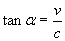,

in which c is the rain’s vertical speed. Light aberration is easily explained once rain drops are compared to light rays and the straw is compared to the telescope. The tilting experimentally measured amounts to 20.5 seconds of arc. If we believe the Earth speed around the Sun to be 30 Km/sec, then the above formula provides a value for c slightly higher than 300,000 Km/sec. More refined measurements of the aberration angle and the Earth speed yields closer estimates to c’s commonly accepted value. Again, similarly to what has happened with Rømer’s method, the tilting of the telescope would seem to provide a way of measuring the one-way speed of light, independently from clocks and clock synchronization. A better look at the formula provided to compute c, though, soon reveals that another speed, the Earth’s one, is required to carry out the actual calculation. As any other velocity, this is measured using clocks and, as we know, these are subject to all the synchronization or time dilation problems previously described. If v is measured using clocks that have been synchronized using the two-way velocity of light, it is not surprising to discover that the velocity of light calculated using the above formula is equivalent to the two-way velocity.

Conclusions

Other methods to measure c have been devised and carried out in past years. Of particular interest seem to be those methods dealing with light frequency and wavelength. The product of these two physical quantities is known to equal the velocity of light. Frequency poses no synchronization problems, as it is normally measured using one clock. Things are different for the wavelength, though. One wavelength can be measured only by having two synchronized clocks at its extremes. This, again, is equivalent to the synchronization of distant clocks, where knowledge of one-way velocity is required. All modern methods to extract the one-way speed of light are, in fact, measuring the two-way velocity.

Will physicists ever be able to overcome this huge impasse and eventually produce an adequate method to measure the one-way velocity of light? There have been very many attempts to formulate new synchronization procedures or, even, devise new experimental techniques aimed at measuring c indirectly. Up to the present day no final word seems to have been pronounced on the subject. Perhaps the most interesting afterthought made on the whole matter is that, Special Relativity being a cornerstone of modern Physics, the majority of successfully tested recent theories do not seem to be touched by the problem. If, ever, someone will come one day with the final solution to this extraordinary challenge, it will for sure have a revolutionary effect on the whole of Physics.